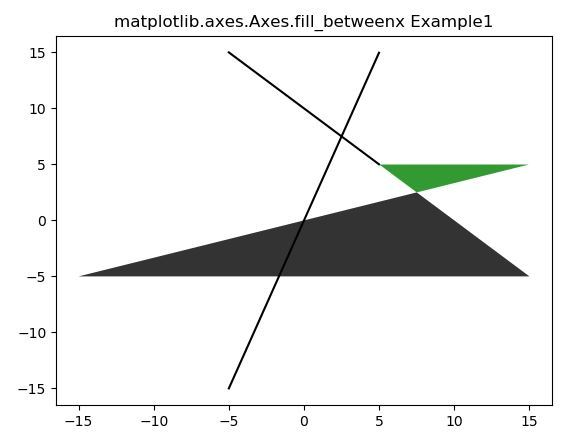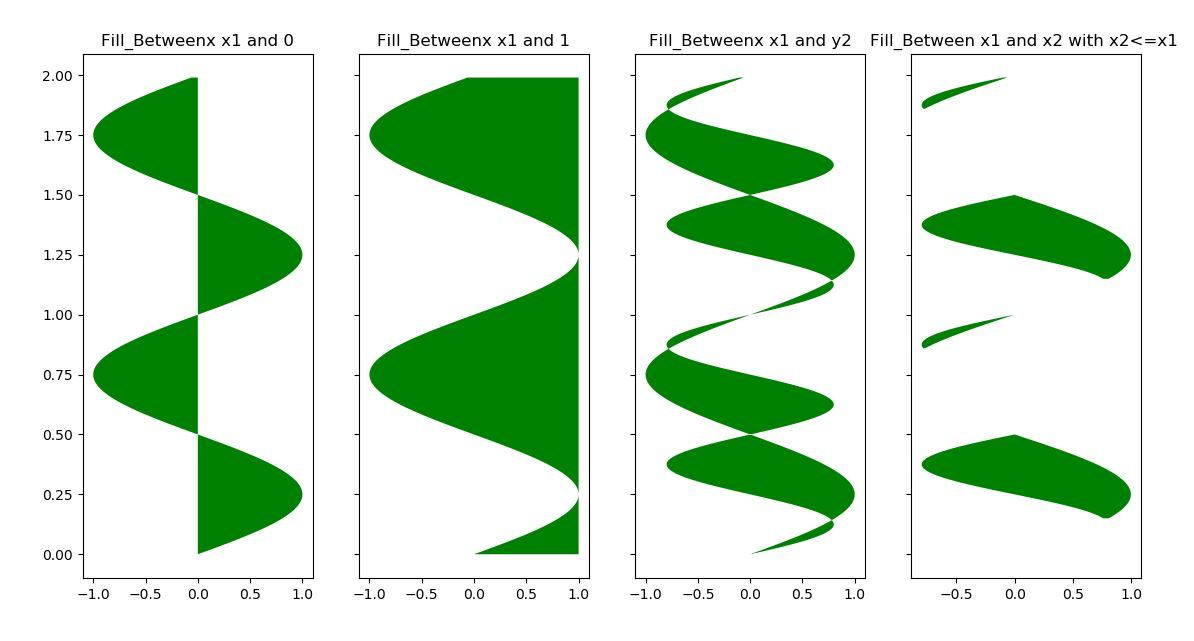# matplotlib.axes.Axes.fill_betweenx() in Python

Matplotlib is a library in Python and it is numerical – mathematical extension for NumPy library. The Axes Class contains most of the figure elements: Axis, Tick, Line2D, Text, Polygon, etc., and sets the coordinate system. And the instances of Axes supports callbacks through a callbacks attribute.

## matplotlib.axes.Axes.fill_betweenx() Function

The Axes.fill_betweenx() function in axes module of matplotlib library is used to fill the area between two vertical curves.

Syntax: Axes.fill_betweenx(self, y, x1, x2=0, where=None, step=None, interpolate=False, *, data=None, **kwargs)

Parameters: This method accept the following parameters that are described below:

• y: This parameter contains the y coordinates of the data points which are used to define the curves.
• x1: This parameter contains the x coordinates of the data points which are used to define the first curves
• x2: This parameter contains the x coordinates of the data points which are used to define the second curves. It is an optional with default value of 0.
• where: This parameter is an optional parameter. And it is used to exclude some horizontal regions from being filled.
• interpolate: This parameter is also an optional parameter. And it is the linewidth of the errorbar lines with default value NONE.
• step: This parameter is also an optional parameter. And it is used to define if the filling should be a step function or not.

Returns: This returns a PolyCollection containing the plotted polygons.

Below examples illustrate the matplotlib.axes.Axes.fill_betweenx() function in matplotlib.axes:

Example-1:

 `# Implementation of matplotlib function ` `      `  `import` `numpy as np ` `import` `matplotlib.pyplot as plt ` `  `  `y ``=` `np.arange(``-``5``, ``5``, ``0.01``) ` `x1 ``=` `-``y ``*` `2` `+` `y ``+` `10` `x2 ``=` `2` `*` `y ``+` `y ` `  `  `fig, ax ``=` `plt.subplots() ` `ax.plot(y, x1, y, x2, color ``=``'black'``) ` `ax.fill_betweenx(y, x1, x2, where ``=` `x2 >x1,  ` `                 ``facecolor ``=``'green'``, alpha ``=` `0.8``) ` ` `  `ax.fill_betweenx(y, x1, x2, where ``=` `x2 <``=` `x1, ` `                 ``facecolor ``=``'black'``, alpha ``=` `0.8``) ` `  `  `ax.set_title(``'matplotlib.axes.Axes.fill_betweenx Example1'``) ` `plt.show() `

Output:Example-2:

 `# Implementation of matplotlib function ` `     `  `import` `numpy as np ` `import` `matplotlib.pyplot as plt ` ` `  `y ``=` `np.arange(``0.0``, ``2``, ``0.01``) ` `x1 ``=` `np.sin(``2` `*` `np.pi ``*` `y) ` `x2 ``=` `0.8` `*` `np.sin(``4` `*` `np.pi ``*` `y) ` ` `  `fig, [ax1, ax2, ax3, ax4] ``=` `plt.subplots(``1``, ``4``, ` `                                         ``sharey ``=` `True``, ` `                                         ``figsize ``=``(``6``, ``6``)) ` ` `  `ax1.fill_betweenx(y, ``0``, x1, facecolor ``=``'green'``) ` `ax1.set_title(``'Fill_Betweenx x1 and 0'``) ` ` `  `ax2.fill_betweenx(y, x1, ``1``, facecolor ``=``'green'``) ` `ax2.set_title(``'Fill_Betweenx x1 and 1'``) ` ` `  `ax3.fill_betweenx(y, x1, x2, facecolor ``=``'green'``) ` `ax3.set_title(``'Fill_Betweenx x1 and y2'``) ` ` `  `ax4.fill_betweenx(y, x1, x2, where ``=` `x2 <``=` `x1,  ` `                  ``facecolor ``=``'green'``) ` ` `  `ax4.set_title(``'Fill_Between x1 and x2 with x2<= x1 '` `) ` `plt.show() `

Output:Whether you're preparing for your first job interview or aiming to upskill in this ever-evolving tech landscape, GeeksforGeeks Courses are your key to success. We provide top-quality content at affordable prices, all geared towards accelerating your growth in a time-bound manner. Join the millions we've already empowered, and we're here to do the same for you. Don't miss out - check it out now!

Previous
Next